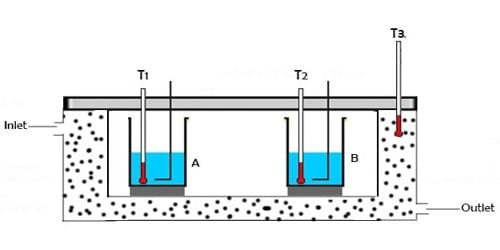Physics

# Explain Fundamental Principle of CalorimetryPrinciple of Calorimetry:

When two bodies of different temperatures are brought in thermal contact then reception and donation of heat take place. The body at higher temperatures leaves heat whereas the body at lower temperatures receives heat. This gives and take will continue until they reach the thermal equilibrium. The body at higher temperature releases heat while the body at lower temperature absorbs heat. The idea that heat energy is a measurement of change in temperature of the body was evolved at a much later time after a series of experiments were conducted using instruments called calorimeters.

Principle: When a hot body is mixed with a cold body, then heat lost by ha is equal to the heat gained by the cold body. If no heat is lost during the donation and reception of heat, then the amount of heat given up by the body at a higher temperature will be equal to the heat gained by the body at a lower temperature.

So, Heat lost = Heat gained

It is called the principle of Calorimetry. The principle of calorimetry indicates the law of conservation energy, i.e. the total heat lost by the hot body is equal to the total heat gained by the cold body.

Key points of Calorimetry:

• Calorimetry is the science of measuring the heat of chemical reactions or physical changes. It involves measurements made with calorimeters.
• The heat of the reaction is the negative of the amount of heat gained by the calorimeter. The calorimeter may be made of more than one component, such as a bomb and a water bath.
• The amount of heat gained by the calorimeter is also a product of the heat capacity of the calorimeter and the change in temperature of the sample undergoing a chemical or physical change.
• When two bodies of dissimilar temperatures (preferably a solid and a liquid) are placed in physical contact with each other, the heat is transferred from the body with higher temperature to the body with lower temperature until thermal equilibrium is attained between them.